2002-11-30

# The Effect Of Array Spacing And The Influence Of The Radiation Pattern Of The Element In A Pattern Synthesis Of Uniform Circular Arrays

## Authors

• Carlos Arturo Suárez Fajardo Universidad Distrital Francisco José de Caldas
• Miguel Ferrando Bataller Universidad Politecnica de Valencia
• Alejandro Valero Nogueira Universidad Politecnica de Valencia

## Keywords:

Síntesis de diagramas de radiación, Agrupaciones circulares, Síntesis con elementos directivos (es).

1

# The Effect of Array Spacing and the Influence of the Radiation Pattern of the Element in a Pattern Synthesis of Uniform Circular Arrays

## C. Suárez

Miembro grupo de investigación en Telecomunicaciones, Universidad Distrital Francisco José de Caldas.

## J. Forero

### Resumen

Este artículo discute el efecto de parámetros como la separación entre elementos en la agrupación y la influencia del diagrama de radiación del elemento utilizado en la síntesis con agrupaciones circulares, con elementos directivos equiespaciados.

Palabras clave: Síntesis de diagramas de radiación, Agrupaciones circulares, Síntesis con elementos directivos.

### Abstract

In this article, the effect of array spacing and the influence of the radiation pattern of the element have been considered in the synthesized radiation pattern using circular arrays of directive elements uniformly arranged.

Key words: Synthesis of radiation patterns, circular arrays, synthesis using directive elements.

### I. INTRODUCTION

This article discusses the synthesis of radiation patterns using circular arrays of directive elements. This type of array is suitable for phased array applications and for multiple beam systems, and it could be also an excellent candidate to face up basestation demands for future wireless communication services.

Fourier series synthesis method will be utilized to synthesize different radiation patterns using circular arrays of N elements with cardioid pattern (ideal elements) and of N dipoles uniformly arranged in front of an infinite metallic cylinder.

### II. PATTERN SYNTHESIS CONSIDERING ELEMENTS WITH CARDIOID PATTERN

Pattern synthesis with uniform circular arrays using elements with cardioid pattern has been studied by several authors -. Most articles focus mainly on sectored patterns, equal side lobes patterns, and gain optimization. In this article, the effect of array spacing and the influence of the radiation pattern of the element have been considered in the synthesized radiation pattern.

Let's begin with a circular array of radius R formed by N+1 directive elements equally spaced. The far field pattern of the array in the plane of the circumference is given by: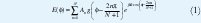where k = 2π/&lambda; , An is the complex excitation of the n th element and g(Φ) = cosn (Φ) denotes the element pattern .

Let Ed be the radiation pattern to be synthesized and bm the Fourier coefficients when the Ed field is expanded in a Fourier series. The excitation coefficients An ,  obtained when equation (1) equals Ed can be expressed as: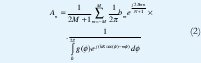By using equations (1) and (2), several simulations have been performed. Some examples are shown in Fig. 1 and Fig. 3b, where a circular array of 9 and 33 ideal directive elements like cardioids with n = 2 have been use to synthesize a radiation pattern, using Taylor, sectored and Chebychev approaches. Fig. 1 shows the effect of the angular separation in the synthesized patterns, for an array with R=0.2149λ and N=9. Notice that for N=9, in this case, d=0.15λ is the maximum separation allowed to obtain accurate synthesized pattern. This fact is specially significant in the case of Taylor.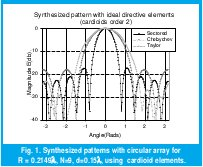Improvement in the synthesized pattern could be obtained if d ≤ 0.15λ, but mutual coupling effects between elements can be increase.

### III. PATTERN SYNTHESIS WITH DIPOLES IN FRONT OF A INFINITE METALLIC CYLINDER

Let's consider now a circular array of radius b, with N half-wavelength dipoles uniformly spaced and arrayed on a circumference in front of an infinite metallic cylinder with radius α and separation of 0.25λ between dipoles and the cylinder. We will estudy the same arrays with N=9 and N=33 dipoles.

The normalized far field radiated by the array in the plane of the circumference , can be expressed as: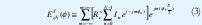where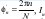, represents the magnitude and phase of the current in each dipole, Ρ=b and Rcr is given by :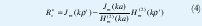The excitation currents In when ETzn (Φ) in (3) equals Ed is given by: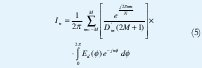where Dm are: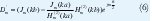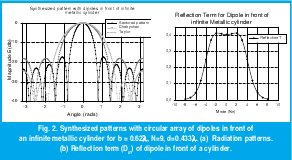Equations (3), (5) and (6) have been used in Fig. 2a and Fig. 3a, where the same synthesized patterns as in section 2 have been carried out with a circular array of 9 and 33 dipoles, disposed on a circle in front of an infinite metallic cylinder (separated 0.25λ) for the cases: b = 0.62λ, d=0.433λ and b = 2.54λ, d=0.484&lambad;. When Fig. 3a and Fig. 3b are compared, it is observed that dipoles provide improved synthesized patterns in all cases when compared with cardioids.

Finally, from (4) and Fig. 2b we can determine the minimal number of modes for the truncation of the infinite series in (3), giving an optimal separation in the range of 0.4λ-0.5λ. In this case Best results can be obtained for elements separation near of 0.5λ.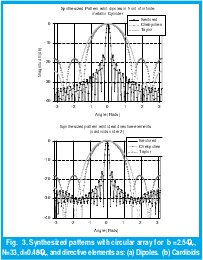### IV. CONCLUSION

We have analyzed circular arrays uniformly spaced using different configurations with cardioid It has been shown that the array spacing required to obtain optimal excitation coefficients (An) is in strong dependence on the pattern of the element used.

### REFERENCES

 B. Sheleg, "A Matrix-Fed circular Array for Continuous Scanning", IEEE, Proc. Vol. 56, No. 11, pp. 2016-2027, November, 1968.

 R. Vescovo, "Use of directional elements in the null synthesis for arc arrays. ". IEEE Trans. Antennas Propagation. Int. symp. Vol. 2. pp .736739, June 1998

 A. W. Rudge, et al. The Handbook of Antenna Design. London: IEE pres. 1986.

 F. I. Tseng, and D. K. Cheng, "Pattern Synthesis of Circular Arrays with Many Directive Elements", IEEE Trans. AP, vol. AP-10, pp. 758-759, Nov. 1968.

 C. Suárez, M. Ferrando y A. Valero, "Radiación de Dipolos Frente a Cilindro Metálico Infinito" URSI Universidad de la Coruña, Septiembre 10-12, 2003.

 C. Suárez, M. Ferrando y A. Valero, "De la síntesis con Agrupaciones Lineales a la síntesis con Agrupaciones Circulares" Revista Ingeniería Universidad Distrital, Vol. 8, No, 1 2003, pp. 46-49.

 C. Suárez, M. Ferrando y A. Valero, "Síntesis con Agrupaciones Circulares" URSI Universidad de la Coruña España, Septiembre 10-12, 2003.

C. Suárez